# Complete regularity is hereditary

This article gives the statement, and possibly proof, of a topological space property (i.e., completely regular space) satisfying a topological space metaproperty (i.e., subspace-hereditary property of topological spaces)
View all topological space metaproperty satisfactions | View all topological space metaproperty dissatisfactions
Get more facts about completely regular space |Get facts that use property satisfaction of completely regular space | Get facts that use property satisfaction of completely regular space|Get more facts about subspace-hereditary property of topological spaces

This article gives the statement, and possibly proof, of a basic fact in topology.

## Statement

Any subset of a completely regular space is completely regular in the subspace topology.

## Definitions used

Term Definition used
completely regular space A space$X$ is completely regular if it is a T1 space and given any point$x \in X$ and closed subset$C \subseteq X$ such that$x \notin C$, there exists a continuous map$f:X \to [0,1]$ such that$f(x) = 0$ and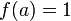$f(a) = 1$ for all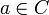$a \in C$.
subspace topology For a subset$A$ of the space$X$, the subspace topology on$A$ is defined as follows: a subset of$A$ is open in$A$ iff it can be expressed as the intersection with$A$ of an open subset of$X$.
Also, a subset of$A$ is closed in$A$ iff it can be expressed as the intersection with$A$ of a closed subset of$X$.

## Related facts

### Similar facts

Property Proof that is is subspace-hereditary
Hausdorff space Hausdorffness is hereditary
regular space regularity is hereditary
Urysohn space Urysohn is hereditary
T1 space T1 is hereditary

Other similar facts:

## Facts used

1. T1 is hereditary

## Proof

Given: A topological space$X$, a subset$A$ of$X$.$X$ is completely regular.

To prove:$A$ is completely regular.

Proof: The T1 property for$A$ follows from Fact (1). It thus suffices to show the separation property for$A$, i.e., that any point and disjoint closed subset in$A$ can be separated by a continuous function. In other words, we want to prove the following.

To prove (specific): For any point$x \in A$ and any closed subset$C$ of$A$ such that$x \notin A$, there exists a continuous function from$A$ to$[0,1]$ such that$f(x) = 0$ and$f(a) = 1$ for all$a \in C$.

Proof (specific):

Step no. Assertion/construction Facts used Given data used Previous steps used Explanation
1 There exists a closed subset$D$ of$X$ such that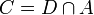$C= D \cap A$ definition of subspace topology$C$ is closed in$A$. Direct from definition. Note: We can, for concreteness, take$D = \overline{C}$, the closure of$C$ in$X$ -- this is the smallest$D$ that works.
2$x$ is not in$D$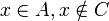$x \in A, x \notin C$ Step (1) [SHOW MORE]
3 There exists a continuous function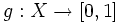$g:X \to [0,1]$ such that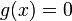$g(x) = 0$ and$g(a) = 1$ for all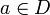$a \in D$.$X$ is completely regular Steps (1), (2) Step-given combination direct
4 Define$f$ as the restriction of$g$ to$A$. In other words, if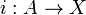$i: A \to X$ is the inclusion mapping, then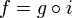$f = g \circ i$. Then,$f$ is a continuous function from$A$ to$[0,1]$. definition of subspace topology (the inclusion mapping from a subset with the subspace topology is continuous), a composite of continuous maps is continuous Step (3) Step-given combination direct
5 The function$f$ defined in Step (4) has the property that$f(x) = 0$ and$f(a) = 1$ for all$a \in C$. Steps (1), (3), (4) The condition on$f$ follows directly from the condition on$g$ in Step (3) and the fact that$C = D \cap A$, so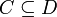$C \subseteq D$.
This proof uses a tabular format for presentation. Learn more about tabular proof formats|View all pages on facts with proofs in tabular format